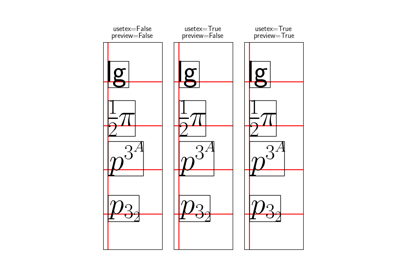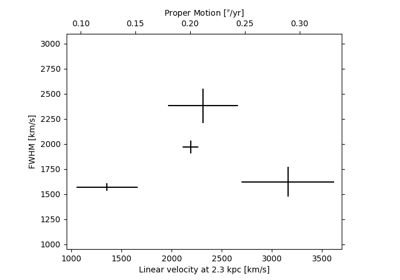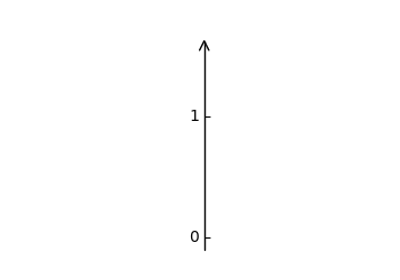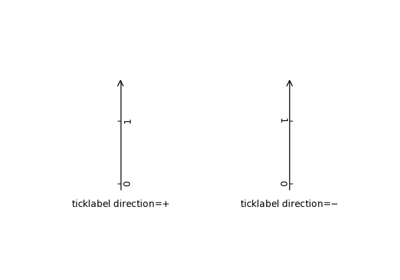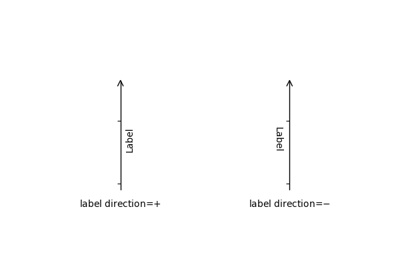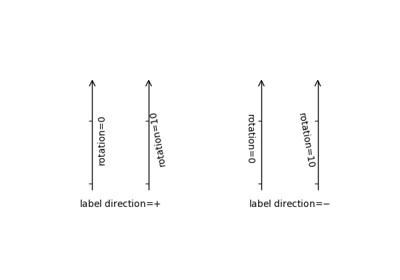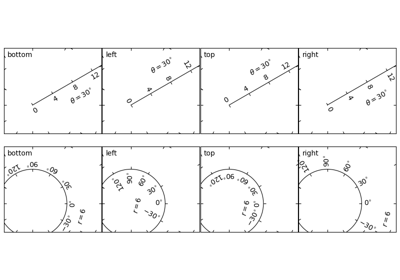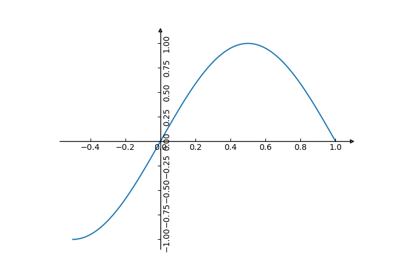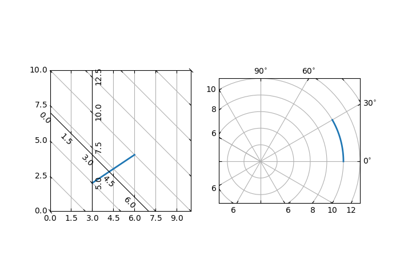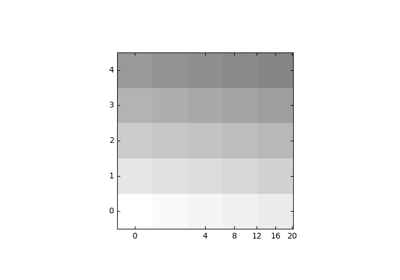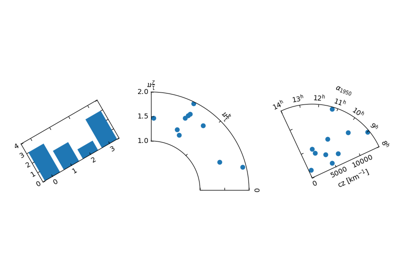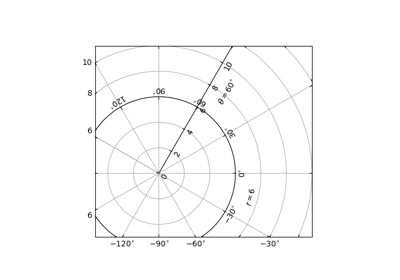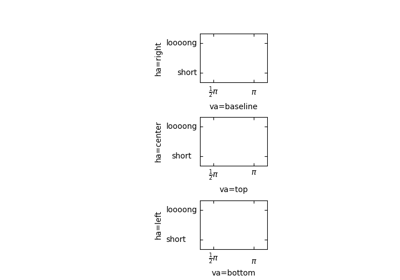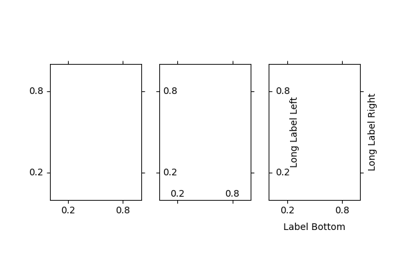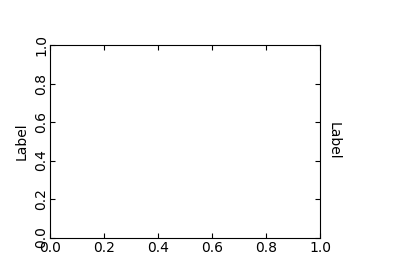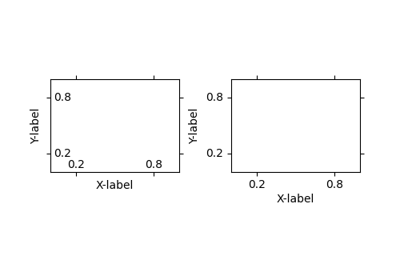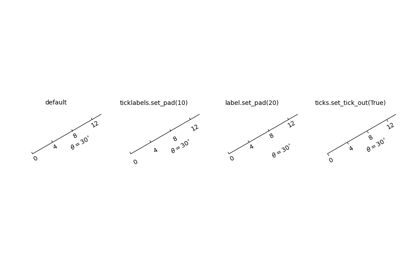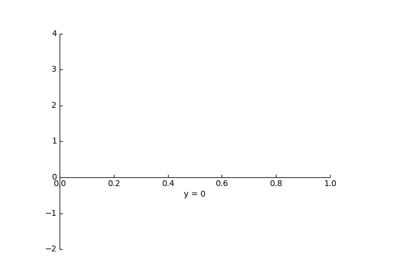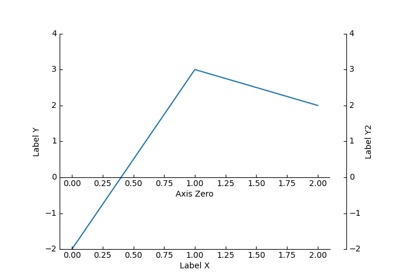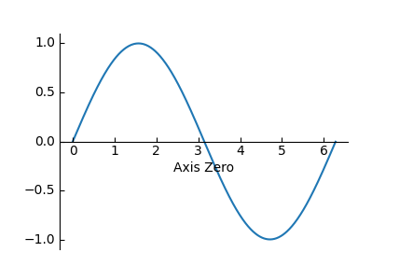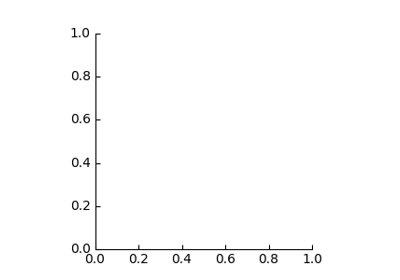matplotlib.axes.SubplotBase¶

class matplotlib.axes.SubplotBase(fig, *args, **kwargs)[source]

Bases: object

Base class for subplots, which are Axes instances with additional methods to facilitate generating and manipulating a set of Axes within a figure.

Parameters: figmatplotlib.figure.Figure *argstuple (nrows, ncols, index) or intThe array of subplots in the figure has dimensions (nrows, ncols), and index is the index of the subplot being created. index starts at 1 in the upper left corner and increases to the right. If nrows, ncols, and index are all single digit numbers, then args can be passed as a single 3-digit number (e.g. 234 for (2, 3, 4)).
change_geometry(self, numrows, numcols, num)[source]

Change subplot geometry, e.g., from (1, 1, 1) to (2, 2, 3).

property colNum
get_geometry(self)[source]

Get the subplot geometry, e.g., (2, 2, 3).

get_gridspec(self)[source]

get the GridSpec instance associated with the subplot

get_subplotspec(self)[source]

get the SubplotSpec instance associated with the subplot

is_first_col(self)[source]
is_first_row(self)[source]
is_last_col(self)[source]
is_last_row(self)[source]
label_outer(self)[source]

Only show "outer" labels and tick labels.

x-labels are only kept for subplots on the last row; y-labels only for subplots on the first column.

property rowNum
set_subplotspec(self, subplotspec)[source]

set the SubplotSpec instance associated with the subplot

update_params(self)[source]

update the subplot position from fig.subplotpars

Examples using matplotlib.axes.SubplotBase¶